Next: Euler's equations Up: Rigid body rotation Previous: Rotational kinetic energy

# Principal axes of rotation

We have seen that, for a general orientation of the Cartesian coordinate axes, the moment of inertia tensor,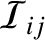, defined in Section 8.3, takes the form of a real symmetric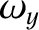matrix. It therefore follows, from the standard matrix theory discussed in Section A.11, that the moment of inertia tensor possesses three mutually orthogonal eigenvectors which are associated with three real eigenvalues. Let the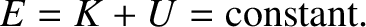th eigenvector (which can be normalized to be a unit vector) be denoted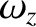, and theth eigenvalue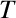. It then follows that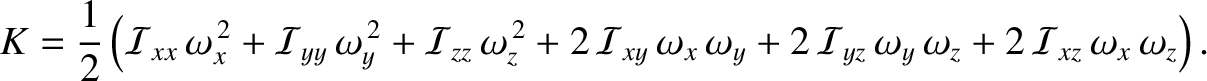(8.19)

for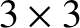.

The directions of the three mutually orthogonal unit vectorsdefine the three so-called principal axes of rotation of the rigid body under investigation. These axes are special because when the body rotates about one of them (i.e., when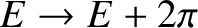is parallel to one of them) the angular momentum vector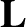becomes parallel to the angular velocity vector. This can be seen from a comparison of Equation (8.13) and Equation (8.19).

Suppose that we reorient our Cartesian coordinate axes so they coincide with the mutually orthogonal principal axes of rotation. In this new reference frame, the eigenvectors ofare the unit vectors,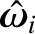,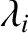, and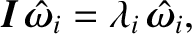, and the eigenvalues are the moments of inertia about these axes,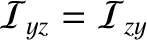,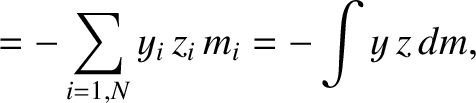, and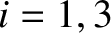, respectively. These latter quantities are referred to as the principal moments of inertia. The products of inertia are all zero in the new reference frame. Hence, in this frame, the moment of inertia tensor takes the form of a diagonal matrix: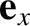(8.20)

Incidentally, it is easy to verify that,, andare indeed the eigenvectors of this matrix, with the eigenvalues,, and, respectively, and that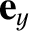is indeed parallel towheneveris directed along,, or.

When expressed in our new coordinate system, Equation (8.13) yields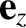(8.21)

whereas Equation (8.18) reduces to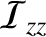(8.22)

In conclusion, there are many great simplifications to be had by choosing a coordinate system whose axes coincide with the principal axes of rotation of the rigid body under investigation.Next: Euler's equations Up: Rigid body rotation Previous: Rotational kinetic energy
Richard Fitzpatrick 2016-03-31